Function Repository Resource:

# HurwitzMatrix

Generate the Hurwitz matrix of a univariate polynomial

Contributed by: Jan Mangaldan
 ResourceFunction["HurwitzMatrix"][poly,x] gives the Hurwitz matrix of poly, treated as a polynomial in x.

## Details

The Hurwitz matrix is also called the Routh-Hurwitz matrix.
The Hurwitz matrix is a matrix constructed from the coefficients of a polynomial, and can be used to check if the polynomial's roots all have negative real parts.
The Hurwitz matrix is a sparse matrix.
In control theory, a polynomial is stable if all of its roots have negative real parts. One can use principal minors of the Hurwitz matrix to make this determination.

## Examples

### Basic Examples (1)

Generate the Hurwitz matrix of a polynomial:

 In:=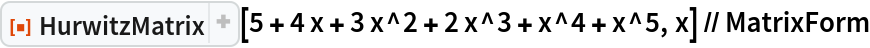Out=### Scope (2)

Generate the Hurwitz matrix from a polynomial with numeric coefficients:

 In:=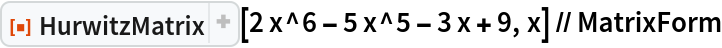Out=Generate the Hurwitz matrix from a polynomial with symbolic coefficients:

 In:=Out=### Applications (2)

Use HurwitzMatrix to check the stability of a polynomial:

 In:=Out=In:=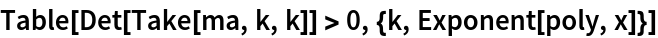Out=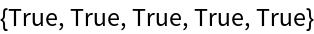Verify stability by computing the roots of the polynomial:

 In:=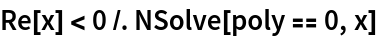Out=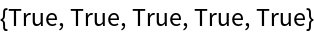### Properties and Relations (4)

A polynomial whose roots all have negative real parts:

 In:=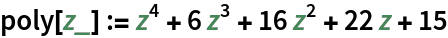The corresponding Hurwitz matrix has principal minors that are all positive:

 In:=Out=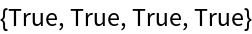Apply a Möbius transformation to the polynomial:

 In:=Out=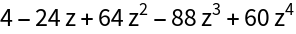The transformed polynomial has a Schur matrix that is positive definite. That is, all of the roots of the transformed polynomial are within the unit disk:

 In:=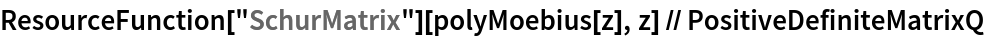Out=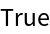## Version History

• 1.1.0 – 27 March 2023
• 1.0.0 – 03 March 2021